## Ratio and Fraction

Practice Unlimited Questions

#### 1. Donald has 125 chickens, 50 turkeys and 25 ducks on his farm. a)   What is the relation between the number of ducks and the number of turkeys? b)   What fraction of the birds on the farm are chickens?

a)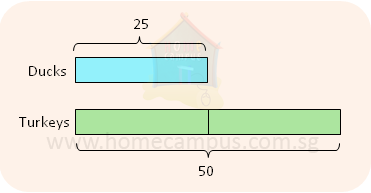In terms of ratio, we say,
The ratio of the number of ducks to the number of turkeys is 1 : 2.

In terms of fraction, we say,
The number of ducks is
 1 2
of the number of turkeys.

Both the things above tell us that,
For every duck on the farm, there are 2 turkeys.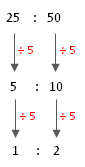b)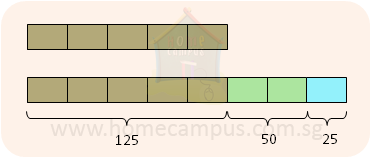125  +  50  +  25  =  200
There are a total of 200 birds on the farm.

125 : 200  =  5 : 8
The ratio of the number of chickens to the number of birds on the farm is 5 : 8.

This means, there are 5 chickens out of every 8 birds on the farm.

In terms of fraction, we say,
 5 8
of the birds on the farm are chickens.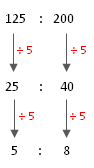#### 2. A certain departmental store rewards shoppers with points for shopping. Alyssa has     1 2 3 as many points as Brendan.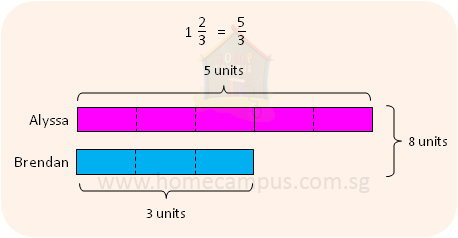a)   What is the ratio of Alyssa's points to Brendan's points?
Alyssa's points : Brendan's points  =  5 : 3
b)   What is the ratio of Brendan's points to Alyssa's points?
Brendan's points : Alyssa's points  =  3 : 5
c)   What is the ratio of Alyssa's points to their total points?
Total points  =  5 units  +  3 units  =  8 units
Alyssa's points : Total points  =  5 : 8
d)   Express Brendan's points as a fraction of their total points.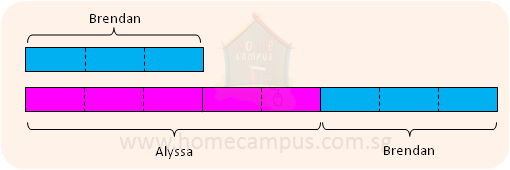Brendan's points are
 3 8
of their total points.
e)   Express Brendan's points as a fraction of Alyssa's points.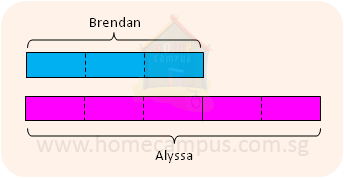Brendan's points are
 3 5
of Alyssa's points.
f)   If they have a total of 72 points, how many points has Alyssa?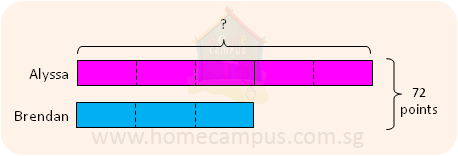8 units  =  72
1 unit   =  9
5 units  =  45

Alyssa has 45 points.

#### 3. Jason has     1 1 2 litres of lemonade and     3 4 litre of cider.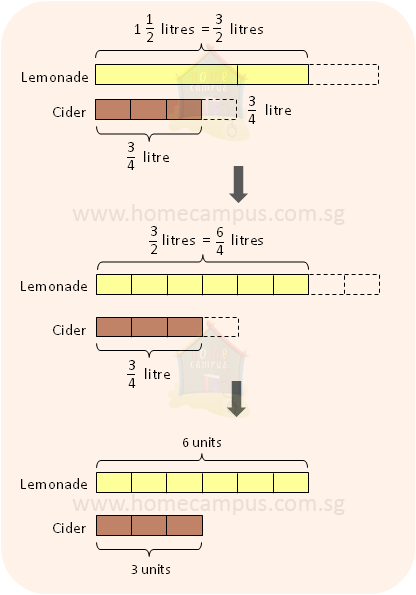a)   How many times as much lemonade as cider does he have?
Quantity of lemonade : Quantity of cider
=  6 : 3
=  2 : 1

He has 2 times as much lemonade as cider.
b)   Express the quantity of cider as a fraction of the quantity of lemonade.
Quantity of cider : Quantity of lemonade
=  3 : 6
=  1 : 2

He has
 1 2